Next: Gauge Invariance Up: Relativity and Electromagnetism Previous: Current Density 4-Vector

# Potential 4-Vector

There are many ways of writing the laws of electromagnetism. However, the most obviously Lorentz invariant way is to write them in terms of the vector and scalar potentials. When written in this fashion, Maxwell's equations reduce to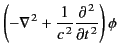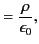(1732)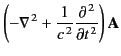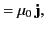(1733)

where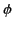is the scalar potential, and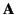the vector potential. Note that the differential operator appearing in these equations is the Lorentz invariant d'Alembertian, defined in Equation (1706). Thus, the previous pair of equations can be rewritten in the form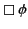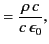(1734)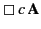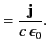(1735)

Maxwell's equations can be written in Lorentz invariant form provided that the entity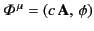(1736)

transforms as a contravariant 4-vector. This entity is known as the potential 4-vector. It follows from Equations (1730), (1734), and (1735) that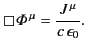(1737)

Thus, the field equations that govern classical electromagnetism can all be summed up in a single 4-vector equation.Next: Gauge Invariance Up: Relativity and Electromagnetism Previous: Current Density 4-Vector
Richard Fitzpatrick 2014-06-27